# How to Print Long Column on One Page in Excel

This post will guide you how to print long columns of data into one page in Excel. How do I split one long column into multiple smaller columns so that you can print it in one page in Excel.

## Print Long Column on One Page

If you want to print one long column into one page in Excel, you need to convert one column into multiple columns in your worksheet. You can use an Excel VBA Macro to convert it quickly. Just do the following steps:

#1 open your excel workbook and then click on “Visual Basic” command under DEVELOPER Tab, or just press “ALT+F11” shortcut.#2 then the “Visual Basic Editor” window will appear.

#3 click “Insert” ->”Module” to create a new module.#4 paste the below VBA code  into the code window. Then clicking “Save” button.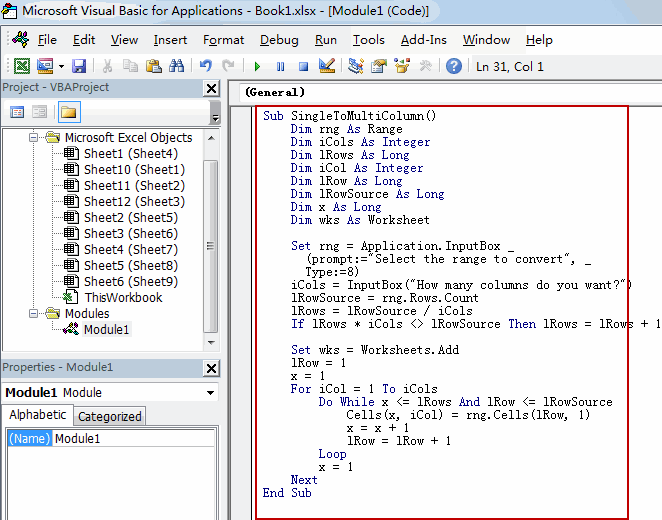```Sub SingleToMultiColumn()
Dim rng As Range
Dim iCols As Integer
Dim lRows As Long
Dim iCol As Integer
Dim lRow As Long
Dim lRowSource As Long
Dim x As Long
Dim wks As Worksheet

Set rng = Application.InputBox _
(prompt:="Select the range to convert", _
Type:=8)
iCols = InputBox("How many columns do you want?")
lRowSource = rng.Rows.Count
lRows = lRowSource / iCols
If lRows * iCols <> lRowSource Then lRows = lRows + 1

lRow = 1
x = 1
For iCol = 1 To iCols
Do While x <= lRows And lRow <= lRowSource
Cells(x, iCol) = rng.Cells(lRow, 1)
x = x + 1
lRow = lRow + 1
Loop
x = 1
Next
End Sub```

#5 back to the current worksheet, then run the above excel macro. Click Run button.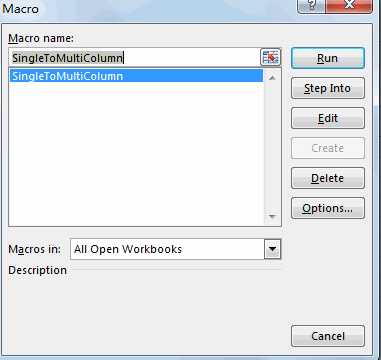#6 Select one range that you want to convert. Click OK button.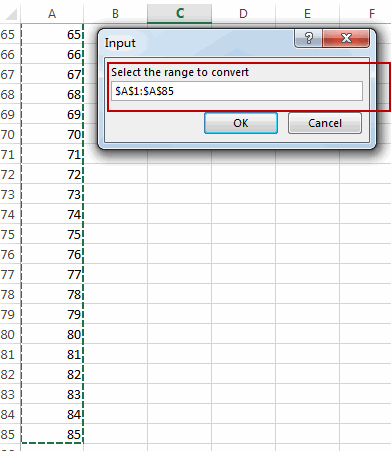#7 Enter one column number that you want to create . Click OK button.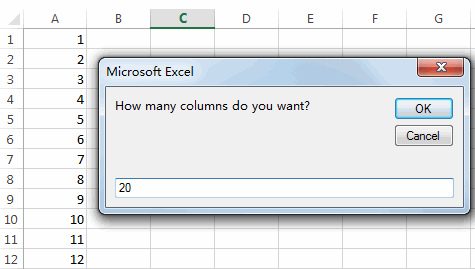#8 Let’ see the result: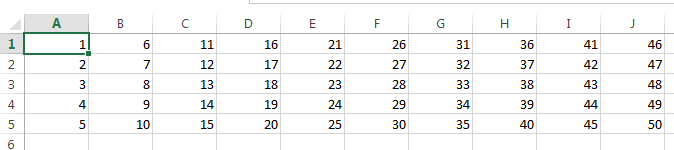Sidebar